## Example Questions

### Example Question #61 : Probability

If a two-sided coin is flipped four times, what is the probability of never getting a head?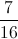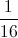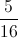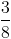Explanation:

The probability of never getting a head is the same as always getting tails. This is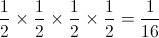.

### Example Question #62 : Probability

If a six-sided die is thrown four times, what is the probability of getting a four everytime?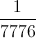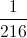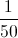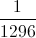Explanation:

The probability is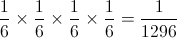.

### Example Question #63 : Probability

If there are 9 marbles in a bag, three white, three blue, and three red, what is the probability of selecting three red marbles at random?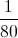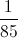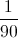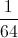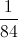Explanation:

The probability of selecting 3 red marbles is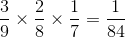.

### Example Question #64 : Probability

There is a box containing 2 red marbles, 7 blue marbles, and an unknown number of green marbles. If the probably of drawing a red marble is 10%, what is the probably that one draws a green marble?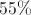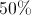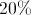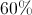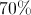Explanation:

Letbe the total number of marbles in the box. Since there's a 10% chance of drawing a red marble, then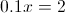implies there are 20 marbles total in the box. Thus, there are only 11 green marbles, resulting in the probability of drawing a green marble being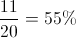### Example Question #65 : Probability

A person rolls a fair 6-sided die twice. What is the probability that the sum of the two rolls is 3?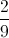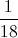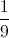Explanation:

In order for the sum to be 3, the two rolls must be 1 and 2. However, it doesn't matter which one comes first. Therefore, there are 2 ways we can acquire the sum being 3. The total number of combination of two die is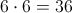.

Thus, the probability of rolling a sum of 3 is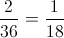.

### Example Question #66 : Probability

A card is drawn randomly from a stardard 52-card deck. What is the probability of drawing a four of hearts?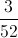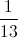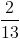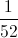Explanation:

The question is asking for the probability of drawing a 4 AND a Heart.

Prob(draw a 4)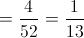.

Prob(draw a Heart)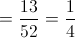.

Then the Prob(4 AND Heart) = Prob(4) * Prob(Heart)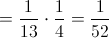You can also solve this question using logic instead of the rules of probability. We know there is only one four of hearts in a deck of cards, so the probability of drawing one must be.

### Example Question #67 : Probability

How many different ways can 15 runners receive ribbons in a race if 1st, 2nd, 3rd, and 4th place ribbons are given?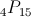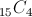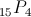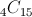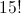Explanation:

Order matters, so a permutation of 15 things chosen 4 at a time, or, is the correct answer.

### Example Question #231 : Data Analysis

A bag of marbles consists of 13 green, 15 blue, 12 black and an unknown number of red marbles. If the chance of choosing a red marble is 1 in 3, how many marbles are there total?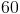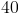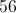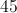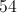Explanation:

Adding the green, blue, and black marbles together gives 40. Then if there is achance that a red marble is drawn, there must be an additional 20 marbles.

### Example Question #232 : Data Analysis

Tiffany picks a peice of fruit from a bowl at random.  The bowl contains 2 red delicious apples, 3 granny smith apples, 1 orange and 3 grapefruits.  What is the probability that she chooses an apple?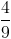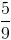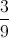Explanation:

The chance she picks an apple is the total number of apples over the total number of fruits in the bowl.  Since there are 2 kinds of apples, we add 2 red delicious and 3 granny smiths to get 5 apples total.  In addition there are 4 more peices of fruit, so the total number of fruits is 9.

### Example Question #233 : Data Analysis

In a bag, there are 6 black marbles and 8 yellow marbles. What is the possibility of choosing a yellow marble at random?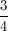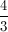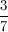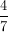There is a total of 14 marbles, of which, 8 are yellow; therefore the probability of choosing a yellow marble is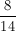or